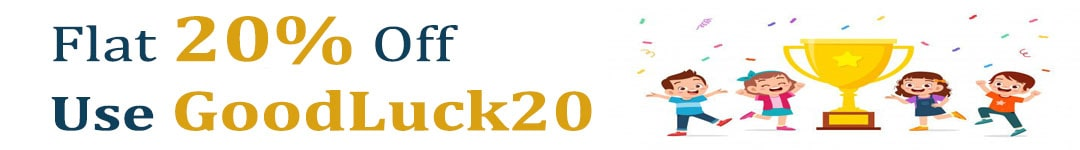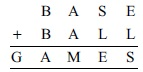# User ForumSubject :IMO    Class : Class 8

Let E = 3, B = 7 and A = 4. Find the other digits in the sum given below out of the given options.AG = 1, S = 3, L = 0, M = 8
BG = 1, S = 0, L = 3, M = 8
CG = 2, S = 3, L = 0, M = 8
DG = 0, S = 3, L = 0, M = 8